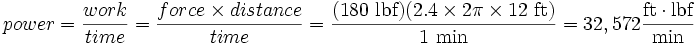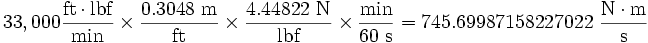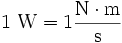Deutsche Versionprefixes | length | area | volume | weight | pressure | temperature | time | energy | density | velocity | acceleration | force

Conversions of power units
Power conversion P
Power P is the quotient of done work W = energy and the taken time t
P = W / t; W = work, t = time. The SI unity of power is watt W
 milliwatts (mW): watts W: pferdestaerke (PS): kilowatts (kW): megawatts (MW): horsepowers (HP):
 W kW kcal/s kcal/h kgf m/s PS HP 1 W = Nm/s = J/s 1 0.001 2.39×10-4 0.860 0.102 0.00136 0.001359 1 kW = 1000 1 0.239 860 102 1.36 1.3596 1 kcal/s = 4190 4.19 1 3600 427 5.69 5.685 1 kcal/h = 1.16 0.00116 0.00028 1 0.119 0.00158 0.001606 1 kgf m/s = 9.81 0.00981 0.00234 8.43 1 0.0133 0.01335 1 PS = 736 0.7360 0.176 623 75 1 0.98632 1 HP = 735.5 0.7355 0.1759 622.577 74.905 1.01387 1

Conversions of many more power units

Calculation: electrical voltage, current, resistance, and power

Power conversion chart

 To convert from: to: multiply by erg per second (erg/s) watt W 1.0×10-7 foot pound-force per hour (ft·lbf/h) watt W 3.766161×10-4 foot pound-force per minute (ft·lbf/min) watt W 2.259697×10-2 foot pound-force per second (ft·lbf/s) watt W 1.355818 horsepower (550 ft·lbf/s) HP or BHP watt W 745.699872 W horsepower (boiler) watt W 9809.50 horsepower (electric) watt W 746 horsepower (metric) = 75 kgf·m/s watt W 735.4987 horsepower (UK) HP or Break HP watt W 745.699872 W horsepower (water) watt W 746.043

Powerunits conversion chart

Sorted from small to large

 Name of Power Unit, P Symbol Definition Relation to SI unit watt, W watt (SI unit) W ≡ J/s = N·m/s = kg·m²/s³ lusec lusec ≡ 1 L·µmHg/s ≈ 1.333 224×10−4 W foot-pound-force per hour ft lbf/h ≡ 1 ft lbf/h ≈ 3.766 161×10−4 W atmospherecubic centimetre per minute atm ccm ≡ 1 atm × 1 cm³/min = 1.688 75×10−3 W foot-pound-force per minute ft lbf/min ≡ 1 ft lbf/min = 2.259 696 580 552 334×10−2 W atmosphere–cubiccentimetre per second atm ccs ≡ 1 atm × 1 cm³/s = 0.101 325 W BTU (International Table) per hour BTUIT/h ≡ 1 BTUIT/h ≈ 0.293 071 W atmosphere–cubic foot per hour atm cfh ≡ 1 atm × 1 cu ft/h = 0.797 001 244 704 W foot-pound-force per second ft lbf/s ≡ 1 ft lbf/s = 1.355 817 948 331 400 W litre-atmosphere per minute L·atm/min ≡ 1 atm × 1 L/min = 1.688 75 W calorie (International Table) per second calIT/s ≡ 1 calIT/s = 4.1868 W BTU (International Table) per minute BTUIT/min ≡ 1 BTUIT/min ≈ 17.584 264 W atmosphere-cubic foot per minute atm·cfm ≡ 1 atm × 1 cu ft/min = 47.820 074 682 24 W square foot equivalent direct radiation sq ft EDR ≡ 240 BTUIT/h ≈ 70.337 057 W litre-atmosphere per second L·atm/s ≡ 1 atm × 1 L/s = 101.325 W horsepower (metric) hp ≡ 75 m kgf/s = 735.498 75 W horsepower (European electrical) hp ≡ 75 kp·m/s = 736 W horsepower (Imperial mechanical) hp ≡ 550 ft lbf/s = 745.699 871 582 270 22 W horsepower (Imperial electrical) hp - ≡ 746 W ton of air conditioning - ≡ 1 t × 1005 J/kg × 1 °F/K ÷ 10 min = 844.2 W poncelet p ≡ 100 m kgf/s = 980.665 W BTU (International Table) per second BTUIT/s ≡ 1 BTUIT/s = 1.055 055 852 62×103 W atmosphere-cubic foot per second atm cfs ≡ 1 atm × 1 cu ft/s = 2.869 204 480 934 4×103 W ton of refrigeration (IT) - ≡ 1BTUIT × 1 sh tn/lb ÷ 10 min/s ≈ 3.516 853×103 W ton of refrigeration (Imperial) - ≡ 1BTUIT × 1 lng tn/lb ÷ 10 min/s ≈ 3.938 875×103 W boiler horsepower bhp ≈ 34.5 lb/h × 970.3 BTUIT/lb ≈ 9.810 657×103 W

 History of the term "horsepower" The term"horsepower" was invented by James Watt to help market his improvedsteam engine. He determined that a horse could turn a mill wheel 144 times in an hour (or 2.4 times a minute). The wheel was 12 feet in radius, thus in a minute the horse travelled 2.4 × 2π × 12 feet. Watt judged that the horse could pull with a force of 180 pounds (just assuming that the measurements of mass were equivalent to measurements of force in pounds-force, which were not well-defined units at the time). So:This was rounded to an even 33,000 ft·lbf/min. Others recount that Watt determined that a pony could lift an average 220 pounds 100 feet (30.48 m) per minute over a four-hour working shift. Watt then judged a horse was 50% more powerful than a pony and thus arrived at the 33,000 ft·lbf/min figure. Engineering in history recounts that John Smeaton initially estimated that a horse could produce 22,916 foot-pounds per minute. John Desaguliers increased that to 27,500 foot-pounds per minute. "Watt found by experiment in 1782 that a 'brewery horse' was able to produce 32,400 foot-pounds per minute". James Watt and Matthew Boulton standardized that figure at 33,000 the next year. Put into perspective, a healthy human can sustain about 0.1 horsepower, and trained athletes can manage up to about 0.3 horsepower for a period of several hours. Most observers familiar with horses and their capabilities estimate that Watt was either a bit optimistic or intended to underpromise and overdeliver; few horses can maintain that effort for long. Regardless, comparison to a horse proved to be an enduring marketing tool. Conversion of historical definition to watts The historical value of 33,000 ft∙bf/min may be converted to the SI unit of watts by using the following conversion of units factors: 1 ft = 0.3048 m 1 lbf = g × 1 lb = 9.80665 m/s2 ×1 lb × 0.45359237 kg/lb = 4.44822 kg·m/s2 = 4.44822 N 60 seconds = 1 minuteAnd the watt is defined asso the historical figure of 33,000 ft·lbf/min converts exactly to the modern definition.

 Break Horsepower is just another way to talk about SAE net horsepower − hp (SAE). Divide DIN 70020 horsepower by 1.0138695680991980 to arrive at SAE net horsepower. 1 PS = 75 kp×m/s = 735.49875 W = 0.9863201652997627 hp (SAE)

 The Power  power = force * distance / time P = F * s / t

 power P: watts force F: newtons distance s: meters time t: seconds
Please enter three values. The forth will be calculated.